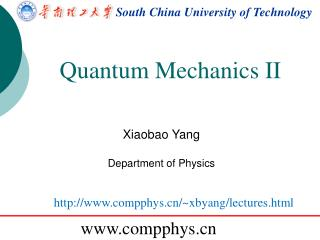DownloadDownload PresentationSouth China University of Technology

# South China University of Technology

Download Presentation## South China University of Technology

- - - - - - - - - - - - - - - - - - - - - - - - - - - E N D - - - - - - - - - - - - - - - - - - - - - - - - - - -
##### Presentation Transcript

1. South China University of Technology Quantum Mechanics II Xiaobao Yang Department of Physics http://www.compphys.cn/~xbyang/lectures.html www.compphys.cn

2. Schrödinger Equation

3. ODE problems • In general, we can classify ordinary differential equations into three major categories: • initial-value problems, which involve time-dependent equations with given initial conditions; • (2) boundary-value problems, which involve differential equations with specified boundary conditions; • (3) eigenvalue problems, which involve solutions for selected parameters (eigenvalues) in the equations. Schrodinger EQ.

4. The shooting method for BVP assume u(0) = u0 and u(1) = u1. Make a guess for u’(0) = α  initial value problem to match u(1) = u1. The idea of the shooting method is to use one of the root search algorithms to find the appropriate α that ensures

5. x=linspace(0,1,100); dx=x(2)-x(1); lamda=linspace(0,100,10000); Without Parity for jj=1:length(lamda) kk=lamda(jj); u=zeros(length(x),1); du=zeros(length(x),1); du(1)=rand; for ii=1:length(x)-1 du(ii+1)=du(ii)-2*kk*u(ii)*dx; u(ii+1)=u(ii)+du(ii+1)*dx; end re(jj)=u(end); end y(0)=rand E(n)=(n*pi)^2/2 plot(lamda,re) hold on plot(lamda,sign(re),'r')

6. y(0) VS lamda clear x=linspace(-5,5,500); dx=x(2)-x(1); V=0.5*x.^2; %V=0.5*(x.^2+0.1*x.^4); lamda=linspace(0,10,1000); dus=linspace(0,1,500); %lamda=-1.8903509 for jj=1:length(lamda) for pp=1:length(dus) kk=lamda(jj); u=zeros(length(x),1); du=zeros(length(x),1); du(1)=dus(pp); for ii=1:length(x)-1 du(ii+1)=du(ii)-2*(kk-V(ii))*u(ii)*dx; u(ii+1)=u(ii)+du(ii+1)*dx; end re(jj,pp)=u(end); end end

7. Searching Eigenvalues with Bisection function y=eigboundary(Es) x=linspace(0,1,100); dx=x(2)-x(1); u=zeros(length(x),1); du=zeros(length(x),1); du(1)=rand; for ii=1:length(x)-1 du(ii+1)=du(ii)-2*Es*u(ii)*dx; u(ii+1)=u(ii)+du(ii+1)*dx; end y=u(end); clear %find x for function y=eigboundary(Es) a=0; b=5; for ii=1:20 ab=0.5*(a+b); if (eigboundary(a)-0)*(eigboundary(ab)-0)<0 b=ab; else a=ab; end end ab

8. Lennar-Jones Problem clear x=linspace(0.5,5,500); dx=x(2)-x(1); V=4*10*((1./x).^12-(1./x).^6); lamda=linspace(-2.2,-1.8,100); %lamda=-1.8903509 for jj=1:length(lamda) kk=lamda(jj); u=zeros(length(x),1); du=zeros(length(x),1); du(1)=rand; for ii=1:length(x)-1 du(ii+1)=du(ii)-2*(kk-V(ii))*u(ii)*dx; u(ii+1)=u(ii)+du(ii+1)*dx; end re(jj)=u(end); end plot(lamda,re) hold on plot(lamda,sign(re)*2*10^20,'r')

9. Matching Method

10. clear x=linspace(-5,5,500); dx=x(2)-x(1); V=0.5*x.^2; %V=0.5*(x.^2+0.1*x.^4); lamda=linspace(0,10,1000); %lamda=-1.8903509 Harmonic oscillator for jj=1:length(lamda) kk=lamda(jj); u=zeros(length(x),1); du=zeros(length(x),1); du(1)=rand; for ii=1:length(x)-1 du(ii+1)=du(ii)-2*(kk-V(ii))*u(ii)*dx; u(ii+1)=u(ii)+du(ii+1)*dx; end re(jj)=u(end); end hold on plot(lamda,re) plot(lamda,sign(re)*max(re),'r')

11. “对应原理” 对应原理是物理学的原理，于1923年被玻尔提出，指在量子數很大而改變很小的情况下，量子理論所得的結果應趨近於經典物理學的結果，反之亦然。

12. Matrix Approach if there are 50 grid elements along both m and n in a two-dimensional problem, you have a 2500 x 2500 matrix to deal with. In three dimensions the matrix would be 125,000 x 125,000.

13. Variational Approach

14. First principle calculations Why we need first principle calculations? What can be calculated? How to do the calculation? How to deal with the results? ……

15. First principle calculations In physics, a calculation is said to be from first principles, or ab initio, if it starts directly at the level of established laws of physics and does not make assumptions such as empirical model and fitting parameters. For example, calculation of electronic structure using Schrödinger's equation within a set of approximations that do not include fitting the model to experimental data is an ab initio approach.

16. 赝势（Pseudo potential）

17. INCAR POTCAR KPOINTS POSCAR KPOINTS Automatic mesh 0 ! number of k-points = 0 ->automatic generation scheme Monkhorst-Pack ! select Monkhorst-Pack (first letter is significant) 1 1 1 ! size of mesh (4x4x4 points along b1, b2, b3) 0. 0. 0. ! shift of the k-mesh INCAR ENCUT = 700 ISMEAR = 0 SIGMA =0.2 NSW = 0 EDIFF = 0.001 POSCAR H2 1.0 12 0 0 0 12 0 0 0 12 2 Cart 0 0 0 0 0 0.75 POTCAR PAW_PBE H 15Jun2001 1.00000000000000000 parameters from PSCTR are: VRHFIN =H: ultrasoft test LEXCH = PE EATOM = 12.4884 eV, .9179 Ry

18. running on 2 nodes distr: one band on 1 nodes, 2 groups vasp.4.6.31 08Feb07 complex POSCAR found : 1 types and 2 ions LDA part: xc-table for Pade appr. of Perdew POSCAR, INCAR and KPOINTS ok, starting setup WARNING: wrap around errors must be expected FFT: planning ... 1 reading WAVECAR WARNING: random wavefunctions but no delay for mixing, default for NELMDL entering main loop N E dE d eps ncg rms rms(c) DAV: 1 -0.469211785182E+01 -0.46921E+01 -0.23142E+02 12 0.981E+01 DAV: 2 -0.738346878115E+01 -0.26914E+01 -0.26914E+01 12 0.252E+01 DAV: 3 -0.741841946701E+01 -0.34951E-01 -0.34951E-01 18 0.423E+00 DAV: 4 -0.741843794358E+01 -0.18477E-04 -0.18477E-04 12 0.955E-02 DAV: 5 -0.741843809963E+01 -0.15606E-06 -0.15606E-06 18 0.878E-03 0.436E+00 DAV: 6 -0.698113710837E+01 0.43730E+00 -0.22006E-01 12 0.179E+00 0.237E+00 DAV: 7 -0.674222335613E+01 0.23891E+00 -0.52473E-01 24 0.281E+00 0.269E-01 DAV: 8 -0.674568380323E+01 -0.34604E-02 -0.54198E-03 12 0.257E-01 0.257E-01 DAV: 9 -0.674122836271E+01 0.44554E-02 -0.74399E-03 12 0.325E-01 0.324E-02 DAV: 10 -0.674397109933E+01 -0.27427E-02 -0.55758E-04 12 0.997E-02 0.187E-02 DAV: 11 -0.674418356913E+01 -0.21247E-03 -0.58782E-05 18 0.303E-02 1 F= -.67441836E+01 E0= -.67441836E+01 d E =-.241108E-10 writing wavefunctions

19. Calculations of H2 molecular

20. Bond energies of H2 436kJ/mol=4.53eV H2 = -6.7715 eV H_atom = -.11048445E+01 eV H-H bond = 4.56 eV

21. Homework Prepare the structural parameters for ordered structures. Sending to 17273799@qq.com when ready For lecture notes, refer to http://www.compphys.cn/~xbyang/